• Category
• >Machine Learning

# Introduction To Principal Component Analysis In Machine Learning

• Rohit Dwivedi
• May 07, 2020
• Updated on: Jun 30, 2021## Introduction

An excess volume of data is expanding widespread in many domains but at the same time interpreting such datasets becomes more difficult. However, in order to extract information from it, various statistical methods have been required to drastically reduce their dimensionality in an appropriate way while making most of the information in the data protected.

Or in simple words, there's a need to lower down feature space to understand the relationship between the variables that will result in fewer chances for overfitting. To reduce or lower down the dimension of the feature space is called “Dimensionality Reduction”. It can be achieved either by “Feature Exclusion” or by “Feature Extraction”.

Many of the techniques have been developed for this purpose where principal component analysis (PCA) is one of the most deployed methods with simple agenda “reducing the dimensionality of a dataset while preserving statistical information as much as possible.

## Principal Component Analysis

Principal component analysis, or PCA, is a dimensionality reduction method that is used to diminish the dimensionality of large datasets by converting a huge quantity of variables into a small one and keeping most of the information preserved.

Specifically, reducing the number of variables can lead to huge loss in accuracy and unavailability of relevant information, therefore PCA works to maintain trade with accuracy and make datasets easier to understand because smaller datasets can be explored and visualized easily as well well analyzing them would be faster for machine learning algorithms without processing additional data variable.Features of PCA, Source

In simplest terms, PCA is such a feature extraction method where we create new independent features from the old features and from a combination of both while keeping only those features that are most important in predicting the target. New features are extracted from old features and any feature can be dropped that is considered to be less dependent on the target variable.

PCA is such a technique that groups the different variables in a way that we can drop the least important feature. All the features that are created are independent of each other.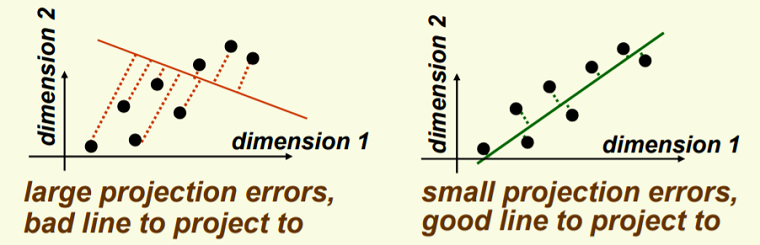Image: Source

Recommended blog: How to use the Random Forest classifier in Machine learning?

In both the pictures above, the data points (black dots) are projected to one line but the second line is closer to the actual points (fewer projection errors) than the first one;

• In the direction of the largest variance, the good line lies, which is used for projection.

• It is needed to modify the coordinate system so as to retrieve 1D representation for vector y after the data gets projected on the best line.

• In the direction of the green line, new data y and old data x have the same variance.

• PCA maintains maximum variances in the data.

• Doing PCA on n dimensions generates a new set of new n dimensions. The principal component takes care of the maximum variance in the underlying data 1 and the other principal component is orthogonal to it that is 2.

Principal component analysis (PCA) is a mainstay of modern data analysis - a black box that is widely used but poorly understood- Source

• Lack of redundancy of data given the orthogonal components.
• Principal components are independent of each other, so removes correlated features.
• PCA improves the performance of the ML algorithm as it eliminates correlated variables that don't contribute in any decision making.
• PCA helps in overcoming data overfitting issues by decreasing the number of features.
• PCA results in high variance and thus improves visualization.
• Reduction of noise since the maximum variation basis is chosen and so the small variations in the background are ignored automatically.

• It is difficult to evaluate the covariance in a proper way.
• Even the simplest invariance could not be captured by the PCA unless the training data explicitly provide this information.
• Data needs to be standardized before implementing PCA else it becomes difficult to identify optimal principal components.
• Though PCA covers maximum variance amid data features, sometimes it may skip a bit of information in comparison to the actual list of features.
• Implementing PCA over datasets leads to transforming actual features in principal components that are linear combinations of actual features, therefore principle components are difficult to read or interpret as compared to actual features.

(Referred blog: What is Confusion Matrix?)

### When to Use PCA?

Case:1 When you want to lower down the number of variables, but you are unable to identify which variable you don't want to keep in consideration.

Case:2 When you want to check if the variables are independent of each other.

Case:3 When you are ready to make independent features less interpretable.

(Must catch: Introduction to Linear Discriminant Analysis

## Principal Component Analysis Mechanism

### 1. Steps for PCA

• Create a correlation matrix or covariance matrix for all the desired dimensions.
• Calculate eigenvectors that are the principal component and respective eigenvalues that apprehend the magnitude of variance.
• Arrange the eigenpairs in decreasing order of respective eigenvalues and pick the value which has the maximum value, this is the first principal component that protects the maximum information from the original data.

### 2. Principal Component Analysis (Performance issues)

• Potency of PCA is directly dependent on the scale of the attributes. PCA will choose the variable that has the highest attributes if they are on a different scale without taking care of correlation.
• PCA can change if the changes are made in variable’s scale.
• Due to the existence of discrete data it can be challenging to interpret PCA.
• Effectiveness of PCA can be influenced by the appearance of skew in the data with long thick tails.
• PCA is unproductive when relationships between attributes are non linear.

### 3. PCA for dimensionality reduction

• PCA is also used for reducing the dimensions.
• According to the respective eigenvalues arrange the eigenvectors in descending order.
• Plot the graph of cumulative eigen_values.
• Eigen vectors that have no importance contributing towards total eigenvalues can be removed for the analysis.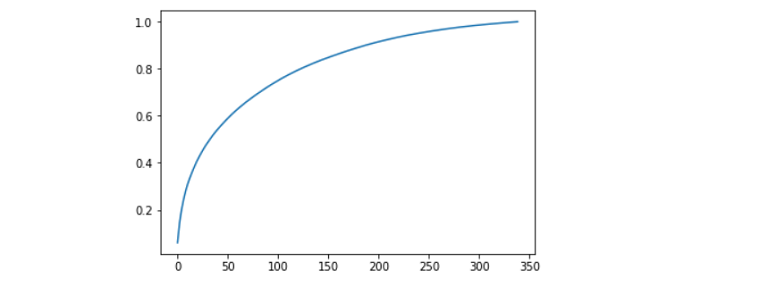Plot of PCA and Variance Ratio

## Principal Component Analysis with Python Code

The dataset on which we will apply PCA is the iris data set which can be downloaded from UCI Machine learning repository.

``````import pandas as pd
import numpy as np
from sklearn.preprocessing import StandardScaler
from sklearn.decomposition import PCA
# importing ploting libraries
import matplotlib.pyplot as plt
from scipy.stats import zscore
from sklearn import datasets
X = iris.data

X_std = StandardScaler().fit_transform(X)
cov_matrix = np.cov(X_std.T)
print('Covariance Matrix \n%s', cov_matrix)``````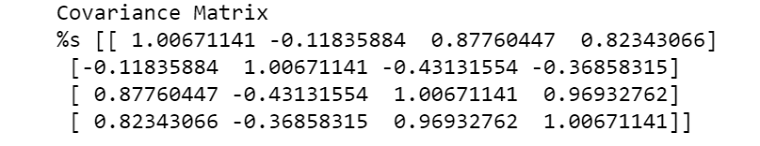Covariance matrix

### STEPS

• Importing the necessary libraries and the dataset.
• Scaling the data using a standard scaler.
• Computing covariance matrix.

``````X_std_df = pd.DataFrame(X_std)
axes = pd.plotting.scatter_matrix(X_std_df)
plt.tight_layout()``````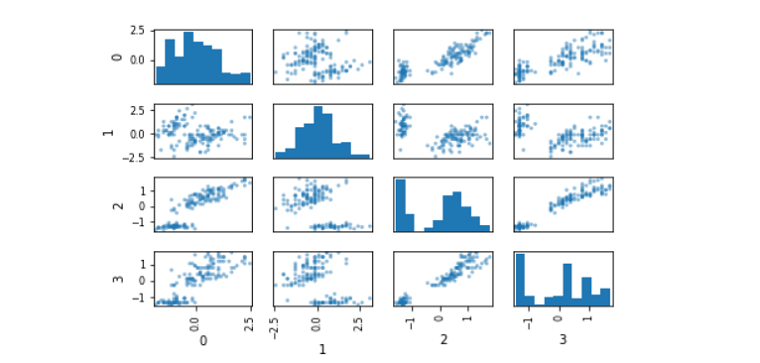Scatter matrix of scaled data

• Plotted scatter matrix of the scaled data.
• Calculated eigenvectors and eigenvalues.

``````eig_vals, eig_vecs = np.linalg.eig(cov_matrix)

eigen_pairs = [(np.abs(eig_vals[i]), eig_vecs[ i, :]) for i in range(len(eig_vals))]
tot = sum(eig_vals)
var_exp = [( i /tot ) * 100 for i in sorted(eig_vals, reverse=True)]
cum_var_exp = np.cumsum(var_exp)
print("Cumulative Variance Explained", cum_var_exp)
``````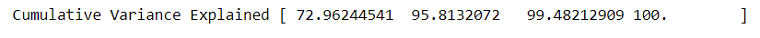``````
plt.figure(figsize=(6 , 4))
plt.bar(range(4), var_exp, alpha = 0.5, align = 'center', label = 'Individual explained variance')
plt.step(range(4), cum_var_exp, where='mid', label = 'Cumulative explained variance')
plt.ylabel('Explained Variance Ratio')
plt.xlabel('Principal Components')
plt.legend(loc = 'best')
plt.tight_layout()
plt.show()
``````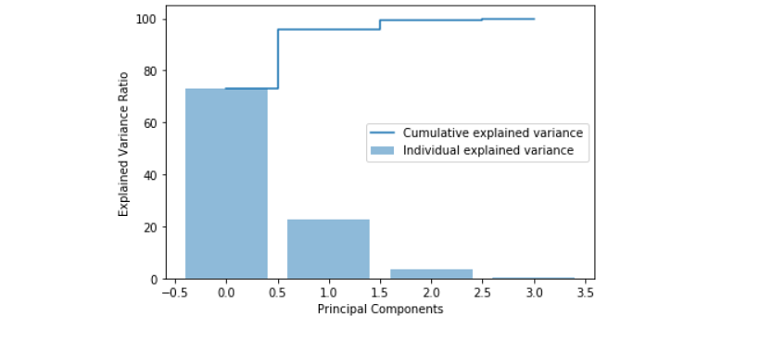Principal components VS variance ratio

First, three principal components explain 99% of the variance in the data, the three PCA will have to be named because they represent a composite of original dimensions. The jupyter notebook file that contains the code of applying PCA on the iris data set can be found here.

(Recommended read: How to use the Random Forest classifier in Machine learning?)

## Conclusion

Now I am ending the blog here by providing the significance of PCA, PCA is a technique that simplifies the complexity of high-dimensional data, maintains variation and extracts strong data trends and patterns in a dataset, it is often used to make data exploration and visualization easier.

With minimal efforts, PCA gives a roadmap over how to cut down complex datasets into lower-dimensional data to obtain hidden yet simplified information.